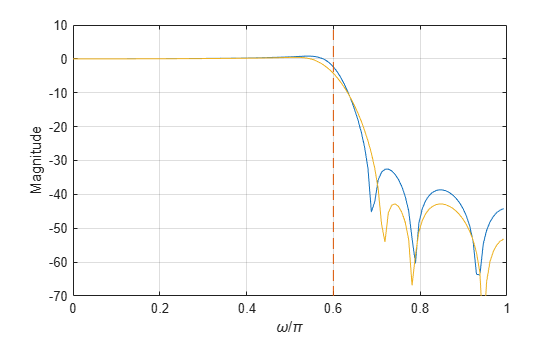# yulewalk

Recursive digital filter design

## Syntax

[b,a] = yulewalk(n,f,m)

## Description

example

[b,a] = yulewalk(n,f,m) returns the transfer function coefficients of an nth-order IIR filter whose frequency magnitude response approximately matches the values given in f and m.

## Examples

collapse all

Design an 8th-order lowpass filter with normalized cutoff frequency 0.6. Plot its frequency response and overlay the response of the corresponding ideal filter.

f = [0 0.6 0.6 1]; m = [1 1 0 0]; [b,a] = yulewalk(8,f,m); [h,w] = freqz(b,a,128); plot(w/pi,mag2db(abs(h))) yl = ylim; hold on plot(f(2:3),yl,'--') xlabel('\omega/\pi') ylabel('Magnitude') gridIncrease the stopband attenuation by specifying a wider transition band.

f = [0 0.55 0.6 0.65 1]; m = [1 1 0.5 0 0]; [b,a] = yulewalk(8,f,m); h = freqz(b,a,128); hold on plot(w/pi,mag2db(abs(h))) hold off ylim(yl)## Input Arguments

collapse all

Filter order, specified as a positive integer scalar.

Data Types: single | double

Frequency points, specified as a vector of points in the range between 0 and 1, where 1 corresponds to the Nyquist frequency, or half the sample rate. The first point of f must be 0 and the last point 1. All intermediate points must be in increasing order. f can have duplicate frequency points corresponding to steps in the frequency response.

Example: [0 0.25 0.4 0.5 0.5 0.7 1] specifies an irregularly sampled Nyquist range.

Data Types: single | double

Magnitude response, specified as a vector containing the desired responses at the points specified in f. m must be the same length as f.

Example: [0 1 1 1 0 0 0] specifies a bandpass magnitude response.

Data Types: single | double

## Output Arguments

collapse all

Filter coefficients, returned as row vectors. The output filter coefficients are ordered in descending powers of z:

$\frac{B\left(z\right)}{A\left(z\right)}=\frac{b\left(1\right)+b\left(2\right){z}^{-1}+\cdots +b\left(n+1\right){z}^{-n}}{a\left(1\right)+a\left(2\right){z}^{-1}+\cdots +a\left(n+1\right){z}^{-n}}.$

## Tips

When specifying the frequency response, avoid excessively sharp transitions from passband to stopband. You may need to experiment with the slope of the transition region to get the best filter design.

## Algorithms

yulewalk designs recursive IIR digital filters using a least-squares fit to a specified frequency response. The function performs the fit in the time domain.

• To compute the denominator coefficients, yulewalk uses modified Yule-Walker equations, with correlation coefficients computed by inverse Fourier transformation of the specified frequency response.

• To compute the numerator, yulewalk follows these steps:

1. Compute a numerator polynomial corresponding to an additive decomposition of the power frequency response.

2. Evaluate the complete frequency response corresponding to the numerator and denominator polynomials.

3. Use a spectral factorization technique to obtain the impulse response of the filter.

4. Obtain the numerator polynomial by a least-squares fit to this impulse response.

 Friedlander, B., and Boaz Porat. "The Modified Yule-Walker Method of ARMA Spectral Estimation." IEEE® Transactions on Aerospace Electronic Systems. Vol. AES-20, Number 2, 1984, pp. 158–173.